## 1. forEach 方法 ​

js
``````let numbers = [1, 2, 3, 4, 5, 4, 3, 2, 1];
numbers.forEach((item, index, array) => {
// 执行某些操作
item += 2
})
console.log(numbers);``````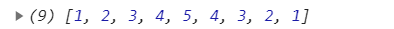js
``````let arr1 = [{
name: 'ljc',
age: 19
}, {
name: 'xy',
age: 18
}]
arr1.forEach(item => {
item.age += 1
})
console.log(arr1);``````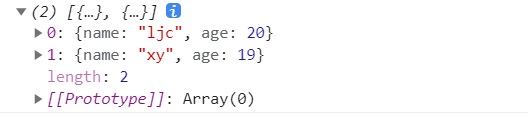## 1-1 手写 forEach 方法 ​

js
``````Array.prototype.myForEach = function (callback, thisArg) {
// 判断调用该API的元素是否为null
if (this == null) {
throw new TypeError('this is null or not defined')
}
// 判断是否为function
if (typeof callback !== "function") {
throw new TypeError(callback + ' is not a function')
}
// 通过this得到调用者arr
const arr = this
// 确定循环变量
let index = 0
// 循环遍历给每个数组元素调用callback
while (index < arr.length) {
// 判断是否存在这个项
if (index in arr) {
// 通过call将this指向thisArg，并且传入3个参数
callback.call(thisArg, arr[index], index, arr)
}
index++
}
}``````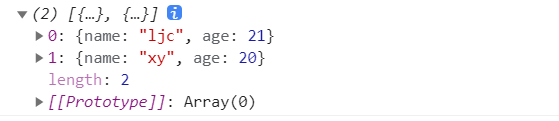## 2. map 方法 ​

`map`也叫映射，也就是将原数组映射成一个新数组

1. 数组中的每一个元素都会调用一个提供的函数后返回结果。
2. 会新建一个数组，需要有承载对象，也就是会返回一个新的对象
3. 除非用原有数组去承载，否则原有数组不会改变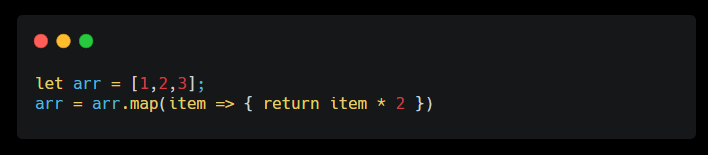js
``````let arr = [1, 2, 3, 4, 5]
let newArr = arr.map(item => item * 2)
console.log(newArr); //  [2, 4, 6, 8, 10]``````

`map`需要有返回值，可以利用箭头函数来简写

`map`中的每一个元素都要执行回调函数，所以必须要有 return，因此不能采用`map`对数组进行过滤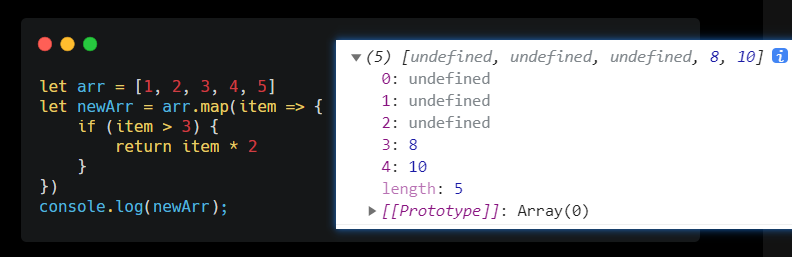## 2-2 手写 map 方法 ​

1. 首先要排除空数组以及没有回调函数的情况
2. 根据`map`的要求需要新建数组，执行函数，返回数组
js
``````Array.prototype.myMap = function (callback, thisArg) {
// 和forEach相同需要进行两个排除
if (this == undefined) {
throw new TypeError('this is null or not defined');
}
if (typeof callback !== 'function') {
throw new TypeError(callback + ' is not a function');
}
// 与forEach不同的是，map会返回一个新数组
const ret = []
// 获得函数调用者
const arr = this
// 数组长度
let len = arr.length
// 对每一个元素执行回调函数
for (let i = 0; i < len; i++) {
// 检查i是否在arr
if(i in arr) {
ret[i] = callback.call(thisArg, arr[i], i, arr)
}
}
// 返回一个处理后的数组
return ret
}``````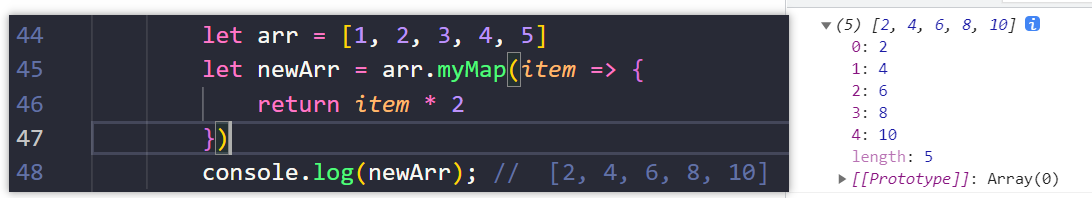## 3. filter ​

`filter`从名字上看可以知道是它是用来做筛选过滤的。和`map`一样，会返回一个新的对象数组，并不会改变原数组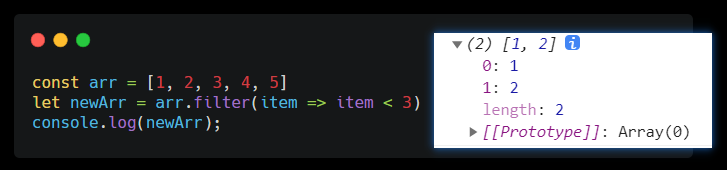## 3-3 手写 filter 方法 ​

`map`方法相比，`filter`需要将满足条件的元素组成新数组返回

js
``````Array.prototype.myFilter = function(callback,thisArg) {
if (this == undefined) {
throw new TypeError('this is null or not defined');
}
if (typeof callback !== 'function') {
throw new TypeError(callback + ' is not a function');
}
// 新数组
const res = []
// 保存this
const arr = this
// 提前计算数组长度
const len = arr.length
for(let i = 0;i<len;i++) {
if(i in arr) {
// 判断元素经过函数调用后，是否有返回值
// 从而来判断是否满足筛选规则，
if(callback.call(thisArg,arr[i],i,arr)) {
res.push(arr[i])
}
}
}
// 最后记得返回新数组噢
return res
}``````

## 4. some 方法 ​

`some`方法用于检查数组中是否有符合条件的值，返回值是个布尔值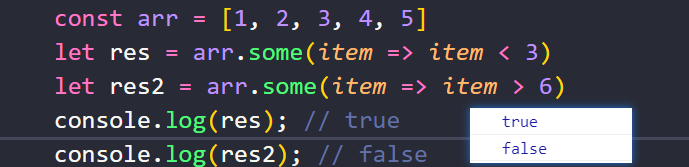`some`方法对于性能来说比较友好，因为不需要全部遍历，只要找到一个符合条件的就会9返回`true`

## 4-4 手写 some 方法 ​

js
``````Array.prototype.mySome = function (callback, thisArg) {
if (this == undefined) {
throw new TypeError('this is null or not defined');
}
if (typeof callback !== 'function') {
throw new TypeError(callback + ' is not a function');
}
let arr = this
let len = arr.length
for (let i = 0; i < len; i++) {
if (i in arr) {
if (callback.call(thisArg, arr[i], i, arr)) {
return true
}
}
}
return false
}``````

## 5. every 方法 ​

`some`相比，每个成员都满足条件才返回`true`，有一个不满足都返回`false`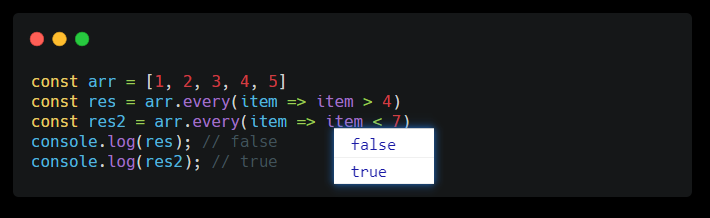## 5-5 手写 every 方法 ​

js
``````Array.prototype.myEvery = function (callback, thisArg) {
if (this == undefined) {
throw new TypeError('this is null or not defined');
}
if (typeof callback !== 'function') {
throw new TypeError(callback + ' is not a function');
}
const arr = this
const len = arr.length
for (let i = 0; i < len; i++) {
if (i in arr) {
if (!callback.call(thisArg, arr[i], i, arr)) {
return false
}
}
}
return true
}``````

## 6. find 和 findIndex 方法 ​

find 使用方法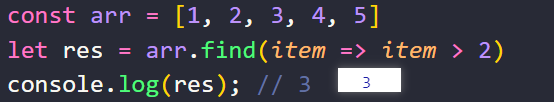findIndex 使用方法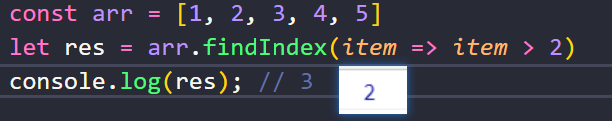## 6-6 手写 find 方法 ​

js
``````Array.prototype.myFind = function (callback, thisArg) {
if (this == undefined) {
throw new TypeError('this is null or not defined');
}
if (typeof callback !== 'function') {
throw new TypeError(callback + ' is not a function');
}
// 保存this，也就是调用者
const arr = this
const len = arr.length
for (let i = 0; i < len; i++) {
if (i in arr) {
if (callback.call(thisArg, arr[i], i, arr)) {
return arr[i]
}
}
}
return undefined
}``````

findIndex 方法

## 小场景 ​

js
``````let staff = [
{name: 'a', salary: 20000, age: 36},
{name: 'b', salary: 19000, age: 34},
{name: 'c', salary: 18000, age: 20},
{name: 'd', salary: 17000, age: 18}
]``````

🤵老板 ：“今年业绩表现不错，所有员工工资涨1000”

👨‍🦲程序员小哥：“简单，用 forEach 就可以了，代码和结果像下面这样”

js
``staff.forEach(item => item.salary += 1000)``

🤵老板：“给我整理成一份工资表格”

👨‍🦲程序员小哥：“没问题，map 有返回值，可以用 map”

js
``````w = staff.map(item => item.salary += 1000)
console.log(w) // [21000, 20000, 19000, 18000]``````

🤵老板：“公司成立这么多年了，给我一份我们公司33岁以上的员工名单吧”

👨‍🦲程序员小哥：“好的，没问题，用filter”

js
``w = staff.filter(item => item.age > 33)``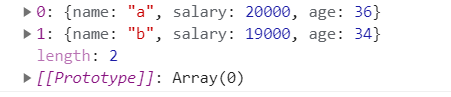👨‍🦲程序员小哥：“a,b员工年龄都33岁以上了”

🤵老板：“那你再帮我看看有没有18岁以下的员工”

👨‍🦲程序员小哥：“好的，用some方法看了一下，我们没有年龄小于18岁的员工”

js
``w = staff.some(item => item.age < 18) // false``

🤵老板：“公司现在上市了，你看看我们公司员工工资是不是都1.6w以上”

👨‍🦲程序员小哥：“真不错啊，全都1.6w以上了，还有什么需要吗？”

js
``w = staff.every(item => item.salary > 16000) // true``

🤵老板：“那你再帮我找个年龄35岁以上的吧，第一个就好”

👨‍🦲程序员小哥：“简单查了一下第一个35以上的，叫a”

js
``w = staff.find(item => item.age > 35) // {name: "a", salary: 20000, age: 36}``

🤵老板：“它在公司的员工数据里排在第几个呀”

👨‍🦲程序员小哥：“你好无聊，这都要看”

js
``w = staff.findIndex(item => item.age > 35) // 0``

👨‍🦲程序员小哥：“0，第一个，元老级别”

🤵老板：“挺不错的，你技术不错嘛，那你把工资总和算出来，叫秘书打钱给财务吧”

👨‍🦲程序员小哥：“....稍等，我再学一下 `reduce`

## 7. reduce 方法 ​

1. 不断地对数组地前两项取出，对它执行目标函数，计算得到的返回值
2. 把返回值插到数组首部，也就是作为`ayyay`
3. 持续执行这个过程，直至数组中的每一项都访问一次
4. 返回最终结果

js
``````const arr = [1, 2, 3]
const res = arr.reduce((prev, cur) => prev + cur)
console.log(res); // 6``````

``````[1, 2, 3] // 取出 1 和 2 ，执行 1 + 2 填回 3
[3, 3] // 取出 3 3 ，填回 6
 // 最终返回6``````

## 7-7 手写 reduce 方法 ​

js
``````Array.prototype.myReduce = function (callback, initialValue) {
// 判断调用该API的元素是否为null
if (this == null) {
throw new TypeError('this is null or not defined')
}
// 判断是否为function
if (typeof callback !== "function") {
throw new TypeError(callback + ' is not a function')
}
const arr = this
const len = arr.length
// 第二个参数
let accumulator = initialValue
let index = 0
// 如果第二个参数是undefined 则数组的第一个有效值
// 作为累加器的初始值
if (accumulator === undefined) {
// 找到数组中的第一个有效值 不一定就是arr
while (index < len && !(index in arr)) {
index++
}
if (index >= len) {
throw new TypeError('Reduce of empty array with no initial value')
}
// 输出第一个有效数组元素，作为累加器的第一个元素
accumulator = arr[index++]
}
while (index < len) {
if (index in arr) {
// arr[index] 为 accumulator 的下一个元素
accumulator = callback.call(undefined, accumulator, arr[index], index, arr)
}
// 持续后移
index++
}
// 返回结果
return accumulator
}``````

## 7-x 利用 reduce 实现 map ​

js
``````Array.prototype.mapReduce = function (callback, context = null) {
if (this == null) {
throw new TypeError('this is null or not defined')
}
// 判断是否为function
if (typeof callback !== "function") {
throw new TypeError(callback + ' is not a function')
}
let arr = this
return arr.reduce((pre, cur, index, array) => {
let res = callback.call(context, cur, index, array)
return [...pre, res]
})
}``````

Last updated: